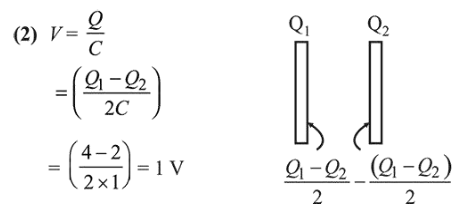# A parallel plate capacitor hasQuestion:

A parallel plate capacitor has $1 \mu \mathrm{F}$ capacitance. One of its two plates is given $+2 \mu \mathrm{C}$ charge and the other plate, $+4 \mu \mathrm{C}$ charge. The potential difference developed across the capacitor is :

1. (1) $3 \mathrm{~V}$

2. (2) $1 \mathrm{~V}$

3. (3) $5 \mathrm{~V}$

4. (4) $2 \mathrm{~V}$

Correct Option: , 2

Solution: